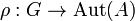# Representation over a category

## Definition

Suppose$G$ is a group and$\mathcal{C}$ is a category. A representation of$G$ over$\mathcal{C}$ is the following data$(A,\rho)$: an object$A \in \operatorname{Ob} \mathcal{C}$, and a homomorphism of groups$\rho:G \to \operatorname{Aut}(A)$.

We are generally interested in studying representations up to equivalence where two representations$(\rho_1,A_1)$ and$(\rho_2,A_2)$ of$G$ are termed equivalent if there exists an isomorphism$\alpha:A_1 \to A_2$ such that, for all$g \in G$:$\alpha \circ \rho_1(g) = \rho_2(g) \circ \alpha$

## Particular cases

Category What a representation over that category is called
category of sets group action or permutation representation
category of vector spaces over a field$K$ linear representations over the field$K$